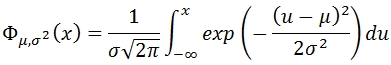# SQL Server NORM.INV function

NORMINV

Updated: 9 August 2010

Use NORMINV to calculate the inverse of the normal cumulative distribution function (cdf). The formula for cumulative distribution function is:Syntax
SELECT [wctStatistics].[wct].[NORMINV] (
<@Probability, float,>
,<@Mean, float,>
,<@Standard_dev, float,>)
Arguments
@Probability
is a probability corresponding to the normal distribution. @Probability is an expression of type float or of a type that can be implicitly converted to float
@Mean
is the arithmetic mean of the distribution. @Mean is an expression of type float or of a type that can be implicitly converted to float
@Standard_dev
is the standard deviation of the distribution. @Standard_dev is an expression of type float or of a type that can be implicitly converted to float
Return Types
float
Remarks
·         If @Probability < 0 or @Probability > 1, NORMINV returns an error
·         If @Standard_dev = 0, NORMINV returns an error
·         If @Mean = 0 and @Standard_dev = 1, NORMINV = NORMSINV(@Probability)
·         NORMINV uses root-finding algorithms and iteration to solve for NORMDIST(NORMINV(@Probability, @Mean, @Standard_dev), @Mean, @Standard_dev,'True') - @Probability = 0to eight decimal places

Examples

Select
wct.NORMINV(0.00173238078965338,10,5)

This produces the following result

----------------------
-4.61589999992911

(1 row(s) affected)

Select
Round(wct.NORMDIST(wct.NORMINV(0.00173238078965338,10,5),10,5,'True')-0.00173238078965338, 9)

This produces the following result

----------------------
0

(1 row(s) affected)Copyright 2008-2020 Westclintech LLC         Privacy Policy        Terms of Service